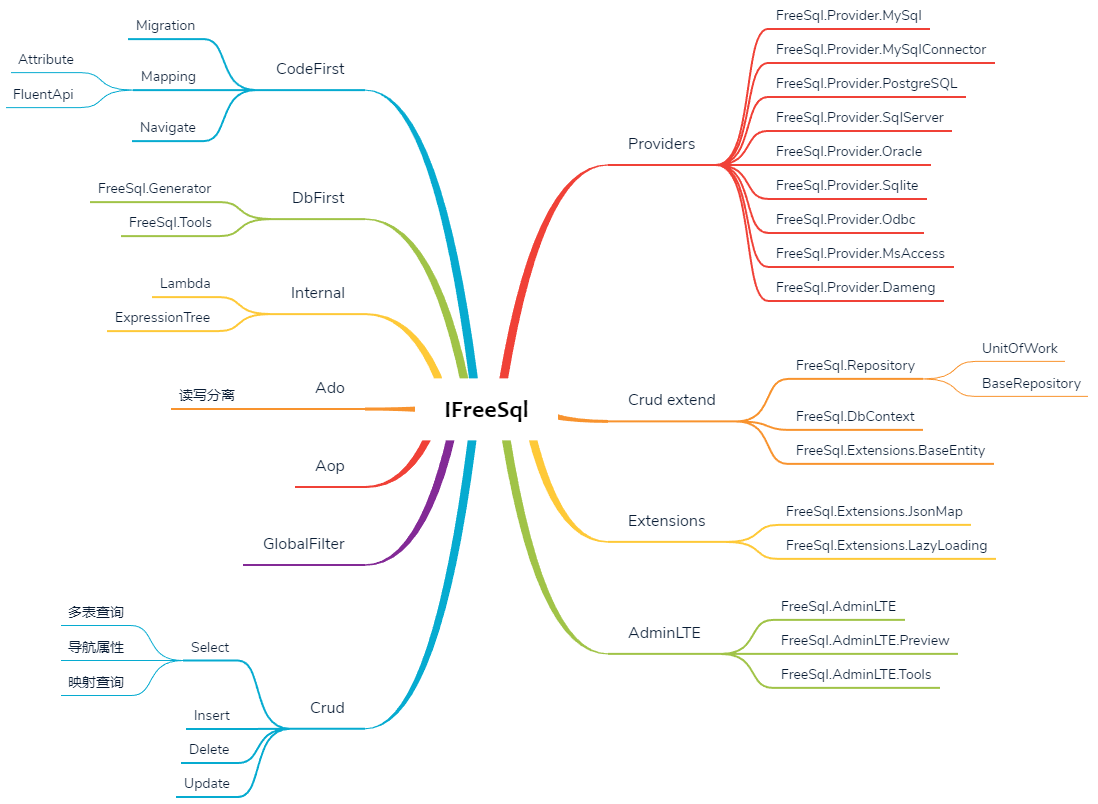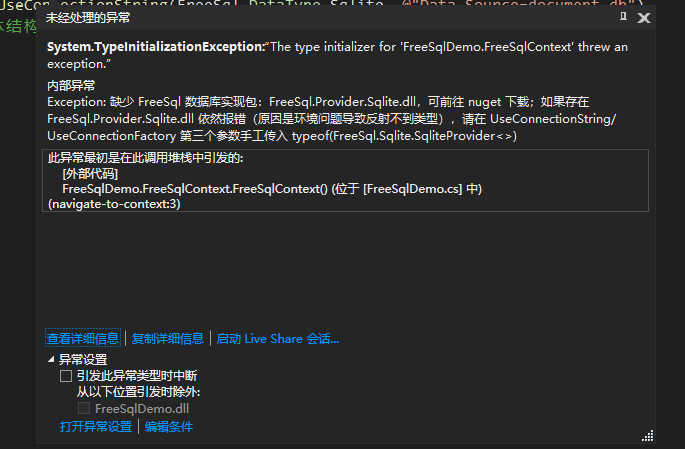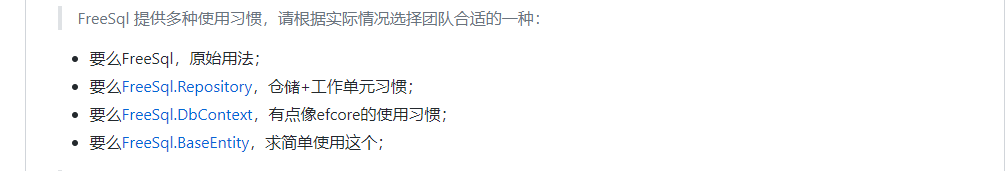# 0. 前言# 1. 初步使用

dotnet add package FreeSql


public class FreeSqlContext
{
public static IFreeSql FreeSqlConnect { get; } =
new FreeSql.FreeSqlBuilder()            .UseConnectionString(FreeSql.DataType.Sqlite, @"Data Source=document.db")
.UseAutoSyncStructure(true) //自动同步实体结构到数据库
.Build();
}


public class Model
{
public int Id { get; set; }
public int StringLength { get; set; }
public string Name { get; set; }
}


## 1.1 简单插入

var row = FreeSqlContext.FreeSqlConnect.Insert(new Model
{
Name = "测试",
StringLength = 10
}).ExecuteAffrows();dotnet add packages FreeSql.Provider.Sqlite


FreeSql针对各种受支持的数据库都单独开发了驱动包，统一命名为：

FreeSql.Provider.<数据库类型>


create table Model
(
Id           INTEGER
primary key,
StringLength INTEGER not null,
Name         NVARCHAR(255)
);


## 1.2 简单查询

var list = FreeSqlContext.FreeSqlConnect.Queryable<Model>().ToList();


## 1.3 简单更新

FreeSql的更新与其他框架相比略显复杂，这里先展示一种更新方式：

list.Name = "修改测试";
row = FreeSqlContext.FreeSqlConnect.Update<Model>().SetSource(list).ExecuteAffrows();


## 1.4 简单删除

row = FreeSqlContext.FreeSqlConnect.Delete<Model>(new[] { list }).ExecuteAffrows();


# 2. 增删改查详解

## 2.1 新增

IInsert<T1> Insert<T1>() where T1 : class;
IInsert<T1> Insert<T1>(T1 source) where T1 : class;
IInsert<T1> Insert<T1>(IEnumerable<T1> source) where T1 : class;
IInsert<T1> Insert<T1>(List<T1> source) where T1 : class;
IInsert<T1> Insert<T1>(T1[] source) where T1 : class;


IInsert<T1> AppendData(T1 source);
IInsert<T1> AppendData(T1[] source);
IInsert<T1> AppendData(IEnumerable<T1> source);


IInsert<T1> IgnoreColumns(string[] columns);
IInsert<T1> IgnoreColumns(Expression<Func<T1, object>> columns);


IInsert<T1> InsertColumns(string[] columns);
IInsert<T1> InsertColumns(Expression<Func<T1, object>> columns);


int ExecuteAffrows();// 返回受影响的列

long ExecuteIdentity();// 返回自增主键值


List<T1> ExecuteInserted();// 返回插入后的数据


## 2.2 删除

IDelete<T1> Delete<T1>() where T1 : class;


IDelete<T1> Delete<T1>(object dywhere) where T1 : class;


主键值 | new[]{主键值1,主键值2} | TEntity1 | new[]{TEntity1,TEntity2} |new{id=1}


IFreeSql中的删除都不会立即删除，都会返回一个IDelete实例，与IInsert一样需要手动调用ExcuteXXX方法。

IDelete<T1> Where(Expression<Func<T1, bool>> exp);
IDelete<T1> Where(string sql, object parms = null);
IDelete<T1> Where(T1 item);
IDelete<T1> Where(IEnumerable<T1> items);


IDelete<T1> WhereDynamic(object dywhere, bool not = false);


FreeSql在设计删除模式时，如果在IFreeSql.Delete中传入参数，后续继续调用Where或者WhereDynamic的话，两次是以and 的形式拼接的条件：

list = FreeSqlContext.FreeSqlConnect.Queryable<Model>().ToList();
FreeSqlContext.FreeSqlConnect.Delete<Model>(list).WhereDynamic(list).ExecuteAffrows();
FreeSqlContext.FreeSqlConnect.Delete<Model>(list).Where(t=>t.Id > 10).ExecuteAffrows();


DELETE FROM "Model" WHERE ("Id" = 1) AND ("Id" = 11)
----------------------------
DELETE FROM "Model" WHERE ("Id" = 1) AND ("Id" > 10)


int ExecuteAffrows();//返回被影响的行数
List<T1> ExecuteDeleted();// 返回被删除的数据，一样只有 Postgresql/SqlServer 有效果


## 2.3 更新

IUpdate<T1> Update<T1>() where T1 : class;
IUpdate<T1> Update<T1>(object dywhere) where T1 : class;


row = FreeSqlContext.FreeSqlConnect.Update<Model>(list).ExecuteAffrows();


IUpdate<T1> UpdateColumns(string[] columns);
IUpdate<T1> UpdateColumns(Expression<Func<T1, object>> columns);
IUpdate<T1> IgnoreColumns(Expression<Func<T1, object>> columns);
IUpdate<T1> IgnoreColumns(string[] columns);


row = FreeSqlContext.FreeSqlConnect.Update<Model>(list).UpdateColumns(new[] { "Name" }).ExecuteAffrows();


IUpdate<T1> SetSource(T1 source);
IUpdate<T1> SetSource(IEnumerable<T1> source);


row = FreeSqlContext.FreeSqlConnect.Update<Model>(list)
.SetSource(list).UpdateColumns(new[] { "Name" }).ExecuteAffrows();
row = FreeSqlContext.FreeSqlConnect.Update<Model>(list)
.SetSource(list).UpdateColumns(new[] { "Name" }).ExecuteAffrows();
row = FreeSqlContext.FreeSqlConnect.Update<Model>(new[] { list ,list})
.SetSource(list).UpdateColumns(new[] { "Name" }).ExecuteAffrows();
row = FreeSqlContext.FreeSqlConnect.Update<Model>(new[] { list, list })
.SetSource(list).UpdateColumns(new[] { "Name" }).ExecuteAffrows();


UPDATE "Model" SET "Name" = @p_0 WHERE ("Id" = 1) AND ("Id" = 1)
--------------------------
UPDATE "Model" SET "Name" = CASE "Id"
WHEN 1 THEN @p_0
WHEN 10 THEN @p_1
WHEN 11 THEN @p_2
WHEN 12 THEN @p_3
WHEN 13 THEN @p_4
WHEN 14 THEN @p_5
WHEN 15 THEN @p_6
WHEN 16 THEN @p_7
WHEN 17 THEN @p_8
WHEN 18 THEN @p_9
WHEN 19 THEN @p_10
WHEN 20 THEN @p_11
WHEN 21 THEN @p_12
WHEN 22 THEN @p_13
WHEN 23 THEN @p_14
WHEN 24 THEN @p_15 END
WHERE ("Id" IN (1,10,11,12,13,14,15,16,17,18,19,20,21,22,23,24)) AND ("Id" = 1)
--------------------
UPDATE "Model" SET "Name" = @p_0 WHERE ("Id" = 1) AND ("Id" = 1 OR "Id" = 10)
--------------------
UPDATE "Model" SET "Name" = CASE "Id"
WHEN 1 THEN @p_0
WHEN 10 THEN @p_1
WHEN 11 THEN @p_2
WHEN 12 THEN @p_3
WHEN 13 THEN @p_4
WHEN 14 THEN @p_5
WHEN 15 THEN @p_6
WHEN 16 THEN @p_7
WHEN 17 THEN @p_8
WHEN 18 THEN @p_9
WHEN 19 THEN @p_10
WHEN 20 THEN @p_11
WHEN 21 THEN @p_12
WHEN 22 THEN @p_13
WHEN 23 THEN @p_14
WHEN 24 THEN @p_15 END
WHERE ("Id" IN (1,10,11,12,13,14,15,16,17,18,19,20,21,22,23,24)) AND ("Id" = 1 OR "Id" = 10)


row = FreeSqlContext.FreeSqlConnect.Update<Model>(list).Set(t => t.StringLength + 1).ExecuteAffrows();


IUpdate<T1> Set<TMember>(Expression<Func<T1, TMember>> exp);
IUpdate<T1> Set<TMember>(Expression<Func<T1, TMember>> column, TMember value);
IUpdate<T1> SetDto(object dto);
IUpdate<T1> SetIf<TMember>(bool condition, Expression<Func<T1, TMember>> exp);
IUpdate<T1> SetIf<TMember>(bool condition, Expression<Func<T1, TMember>> column, TMember value);


• Expression<Func<T1, TMember>> exp 表示在字段本身值的基础上进行操作
• Expression<Func<T1, TMember>> column, TMember value 表示将 column设置 value
• object dto 一个包含要更新属性和值的匿名类，或者一个字典类型（键为要更新的列，值为对应列的值）
• bool condition 表示满足条件则更新，否则将不进行更新

IUpdate也提供了Where模式：

IUpdate<T1> Where(Expression<Func<T1, bool>> exp);
IUpdate<T1> Where(string sql, object parms = null);
IUpdate<T1> Where(T1 item);
IUpdate<T1> Where(IEnumerable<T1> items);
IUpdate<T1> WhereDynamic(object dywhere, bool not = false);


FreeSqlContext.FreeSqlConnect.Update<Model>(list).Set(t => t.StringLength + 1).ExecuteAffrows();
FreeSqlContext.FreeSqlConnect.Update<Model>(list).SetDto(new { Name="测试2" }).ExecuteAffrows();
FreeSqlContext.FreeSqlConnect.Update<Model>(list).SetIf(true, t => t.Name + 1).ExecuteAffrows();
// 或者以下模式
FreeSqlContext.FreeSqlConnect.Update<Model>()
.Where(t => true)
.Set(t => t.StringLength + 1)
.ExecuteAffrows();
// 或者
FreeSqlContext.FreeSqlConnect.Update<Model>(1)
.Set(t => t.StringLength + 1)
.ExecuteAffrows();


int ExecuteAffrows();// 返回受影响的行数
List<T1> ExecuteUpdated();// 嗯， 只有 Postgresql/SqlServer 有效果


## 2.4 查询

FreeSql的查询有两种方式，一种是使用FreeSql的ISelect方法，一种是使用扩展出来的Queryable方法，两者最终返回是一样的，均返回了一个ISelect实例。

ISelect<T1> Select<T1>() where T1 : class;
ISelect<T1> Select<T1>(object dywhere) where T1 : class;
// 扩展方法在  FreeSqlGlobalExtensions 类
public static ISelect<T> Queryable<T>(this IFreeSql freesql) where T : class;


T1 First()
TDto First<TDto>();
TReturn First<TReturn>(Expression<Func<T1, TReturn>> select);
T1 ToOne();
TDto ToOne<TDto>();

• First和ToOne都是返回第一条数据
• TDto 表示要查询出来的字段合集，列名与数据表中一一对应
• Expression<Func<T1, TReturn>> select 类型投影，通过lambda语句建立T1到TReturn之间的关系

List<T1> ToList(bool includeNestedMembers = false);
List<TDto> ToList<TDto>();
List<TReturn> ToList<TReturn>(Expression<Func<T1, TReturn>> select);

• includeNestedMembers ： false: 返回 2级 LeftJoin/InnerJoin/RightJoin 对象；true: 返回所有 LeftJoin/InnerJoin/RightJoin的导航数据

long Count();// 返回数目
ISelect<T1> Distinct();//去重
ISelect<T1> Skip(int offset);// 忽略几个
ISelect<T1> Take(int limit);// 获取前几个
ISelect<T1> OrderBy<TMember>(Expression<Func<T1, TMember>> column);// 排序
ISelect<T1> OrderBy<TMember>(bool condition, Expression<Func<T1, TMember>> column);// 排序
ISelect<T1> OrderByDescending<TMember>(Expression<Func<T1, TMember>> column); // 降序
ISelect<T1> OrderByDescending<TMember>(bool condition, Expression<Func<T1, TMember>> column);// 降序
decimal Sum<TMember>(Expression<Func<T1, TMember>> column);// 求和
double Avg<TMember>(Expression<Func<T1, TMember>> column);// 求平均数


ISelect<T1> Where(Expression<Func<T1, bool>> exp);
ISelect<T1> WhereIf(bool condition, Expression<Func<T1, bool>> exp);
ISelect<T1> Where(string sql, object parms = null);


# 3. 总结

FreeSql可以说结合了很多优秀的ORM框架内容，而且针对不同的方式分成了不同的插件形式，使主干可以轻装上阵。posted @ 2020-05-29 11:46  月影西下  阅读(3249)  评论(10编辑  收藏  举报